## Example Questions

1 3 Next →

### Example Question #21 : Other Percentage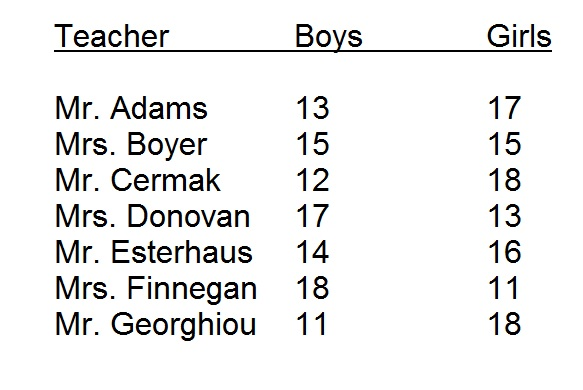Jones Elementary School has seven eighth-grade teachers; each teacher has the number of boys and girls listed above.

What percent of the students in eighth-grade are boys? Choose the closest answer.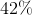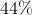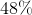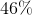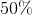Explanation:

The number of boys adds up to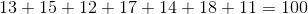The number of girls adds up to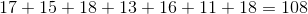The total number of students is therefore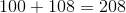.

The percent of the students that are boys is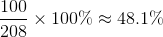,

making 48% the closest choice.

### Example Question #22 : Other Percentage

What percent of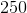is?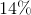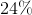Explanation:

We can set up a proportion to solve this problem. Remember that a percentage can be written as a fraction.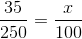Now we can cross-multiply to find our percent.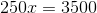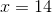Therefore, our answer is.

### Example Question #113 : Percentage

Marisa and Ted are running for senior class president. 150 votes have been tallied so far; 88 for Marisa and 62 for Ted. If 250 students total are voting, how many more votes must Marisa win in order to defeat Ted and earn exactly 50% more votes than him?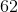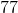Explanation:

For Marisa to earn 50% more votes than Ted, she will need to earn 1.5 times his amount of votes. If we call Ted's amount of votes x, and the total number of votes is 250, we can set up the equation: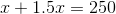If we combine like terms, we get: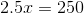And if we solve for x, we get: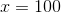This means that Ted will get 100 votes. Since there are 250 votes total, we can figure out that Marisa will end up with 150 votes.

The question asks "how many more votes must Marisa win in order to defeat Ted and earn exactly 50% more votes than him?" and tells us that Marisa already has 88 votes.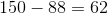, therefore, Marisa needs to earn 62 more votes.

### Example Question #118 : Percentage

What percent of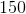is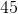?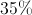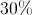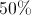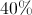Explanation:

This question involves the verbal cues "of" and "is."  "Of" means to multiply and "is" means equal.

Thus the equation to solve becomes: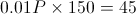.

1 3 Next →

### All SAT Math Resources